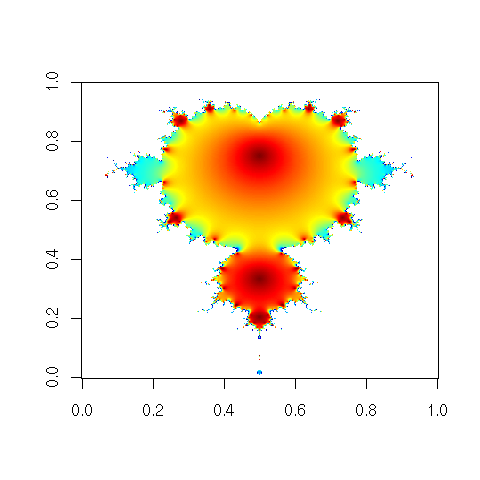DATA MINING
Desktop Survival Guide
by Graham Williams# Animated Mandelbrot

The following example, suggested by Jaroslaw Tuszynski (author of the caTools package), illustrates the use of complex numbers constructed using complex, as well as the writing of multiple images to a gif file to construct an animated gif. Some 160,000 (400 by 400) complex numbers are generated and stored in a matrix. A list of 20 arrays is then built, each being 400 by 400. The magic is then done in the for loop, 20 times, to generate 20 images. All images are written to a single gif file using write.gif. The final image (X[,,k]) is also displayed using image. We use tim.colors from fields to select a pleasing rainbow colour sequence, but replace the first with transparent to achieve a clear background.```library(fields) # for tim.colors library(caTools) # for write.gif m <- 400 # grid size C <- complex(real=rep(seq(-1.8,0.6, length.out=m), each=m), imag=rep(seq(-1.2,1.2, length.out=m), m)) C <- matrix(C, m, m) Z <- 0 X <- array(0, c(m, m, 20)) for (k in 1:20) { Z <- Z^2+C X[,,k] <- exp(-abs(Z)) } col <- tim.colors(256) col <- "transparent" write.gif(X, "graphics/rplot-mandelbrot.gif", col=col, delay=100) image(X[,,k], col=col) # show final image in R ```

http://rattle.togaware.com/code/rplot-mandelbrot.R